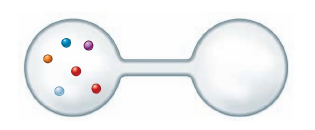# Problem: Consider the following illustration of six molecules of gas in a two-bulbed flask.b. Determine the probability of finding the gas in its most likely arrangement.

⚠️Our tutors found the solution shown to be helpful for the problem you're searching for. We don't have the exact solution yet.

###### Problem Details

Consider the following illustration of six molecules of gas in a two-bulbed flask.b. Determine the probability of finding the gas in its most likely arrangement.